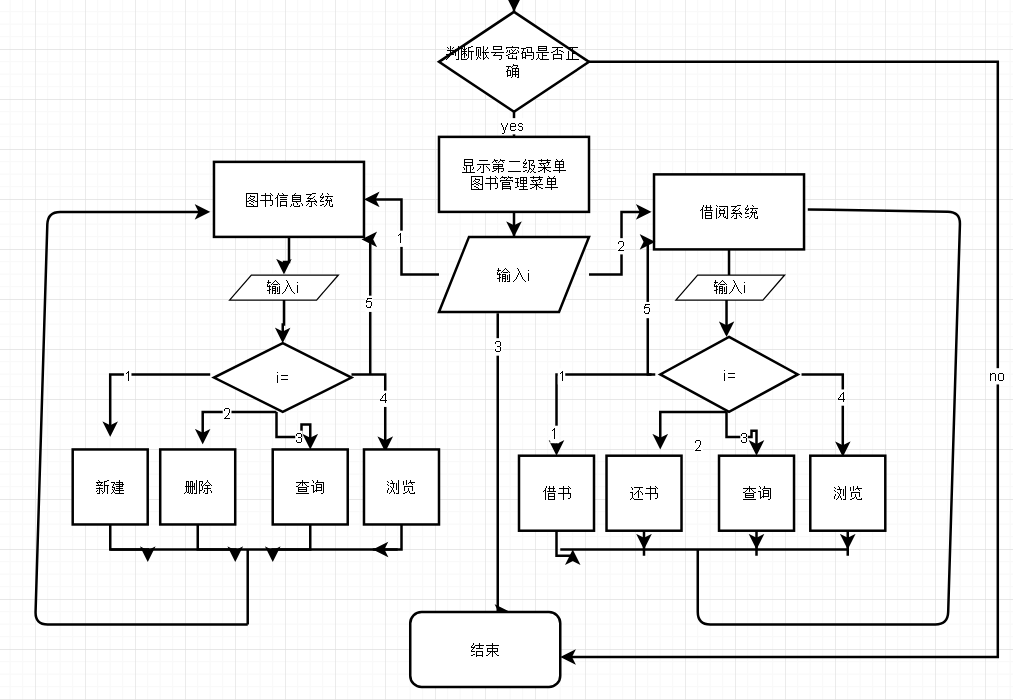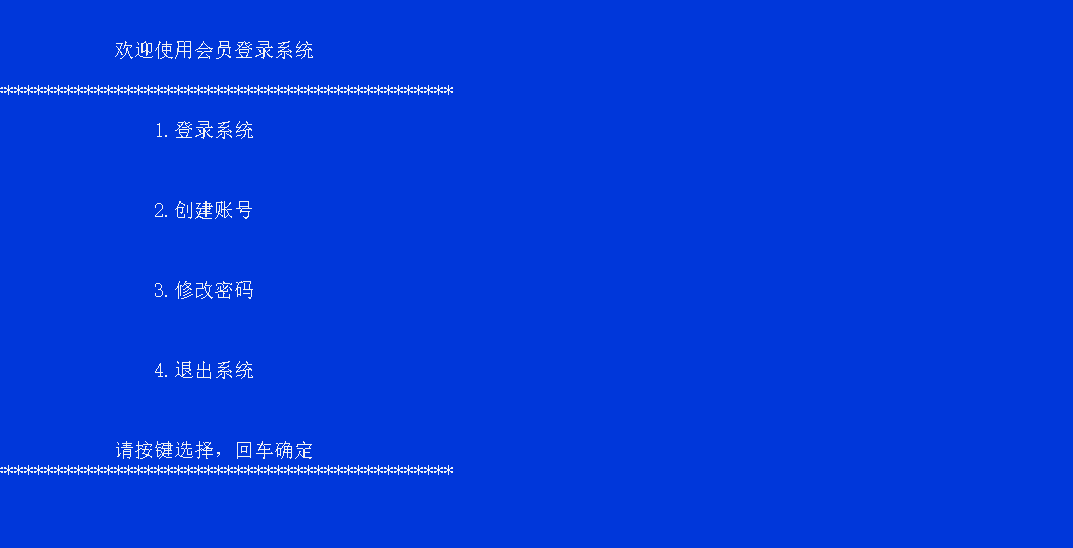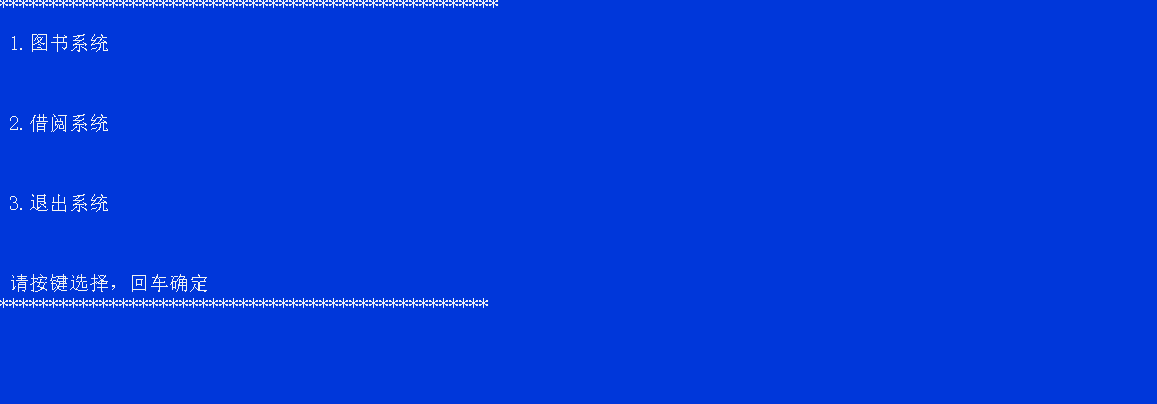• #include <stdio.h> #include <string.h> typedef struct { char name; char pw; }uifo; #define USER_FILE "data.bin" #define DEFAULT_NAME "guest" #define DEFAULT_PW "123" void write_data(uifo *in
• 本次课程设计使用C语言制作程序来实现图书的登记，删除，查询，浏览以及读者的借阅，还书，会员登录，修改密码等功能。程序中涉及到数据链表和文件指针的操作，包括结构体数据定义，使用及文件的读写，定位，修改等...
C课程设计——图书管理系统
1、题目意义
图书馆，作为文献的聚集地和展示平台，常常扮演着引领文化前进的角色，是每个大学不可或缺的基础设施，而图书管理系统则是一个图书馆能够正常运转的关键。本次课程设计使用C语言制作程序来实现图书的登记，删除，查询，浏览以及读者的借阅，还书，会员登录，修改密码等功能。程序中涉及到数据链表和文件指针的操作，包括结构体数据定义，使用及文件的读写，定位，修改等。
2、设计思想
①新建账号密码，然后通过二者的匹配登录系统，这样可以保证是本校同学才能使用，另外还可以修改密码。
②添加图书的基本信息，如 书号，书名，作者，出版社，类别，进库量；
③删除图书，通过图书的书名进行删除；
④图书查询，通过图书的书号，书名，作者或者类别进行检索；
⑤浏览图书的库存，将所有数目展示出来；
⑥借书登记，先判断是否有这本数，然后进行借书人学号，姓名，归还截止期限，借书书名进行登记
⑦还书情况查看。
⑧借阅情况查看。
⑨会员登录，通过数据文件中的已注册的账号密码来登录到图书管理系统。
⑩修改密码
3.总设计框架图4.代码展示#include <stdio.h>
#include <stdlib.h>//其中包含system函数
#include <conio.h>//定义了通过控制台进行 数据输入 和数据输出的函数，如getch函数。
#include <string.h>//定义字符数组
#include <math.h>
#define LEN sizeof(struct book)//有关图书信息的结构体
#define LEN1 sizeof(struct reader)//有关读者信息的结构体
#define LEN2 sizeof(struct land)//有关登录系统的结构体

struct book//图书信息
{
char num,nam,aut,pub,cat;
int  many;
float price;
struct book*next;
};

struct reader//读者借阅信息
{
int jnum;
char jnam,time,tsnam;
struct reader *next;
};
struct land//登录信息
{
int zhanghao;
char password;
struct land*next;
};

int tjzs();//统计library文本个数函数
void xg(int,char[] );
void mainmenu();//显示主菜单
void tsmenu();//显示图书信息菜单
void jmenu();//显示借阅信息菜单
void lmenu();//显示登录菜单

void main1();// 主菜单功能
void tsmain();//图书菜单功能
void cxts();//查询图书
void zjts();//增加图书
void scts();//删除图书
void llts();//浏览图书

void jmain();//读者借阅信息
void js();//借书
void hs();//还书
void cxjs();//查询借书
void lljs();// 浏览借书情况

void land();//登录功能系统
int xinjian(int,char[] );//创建账号密码
void xgmm();//修改密码
void lmain();//登录界面函数

int tjzs()//统计图书文本个数
{
FILE *fp;
int txcl=0,n;
float tprice=0;
char tname={'\0'},tauthor={'\0'},tchuban={'\0'},tkind={'\0'},tshuhao={'\0'};
fp=fopen("library.txt","r");//打开文件
for (n=0;!feof(fp);n++)//逐个读文件
fscanf(fp,"%s%s%s%s%s%d%f",tshuhao,tname,tauthor,tchuban,tkind,&txcl,&tprice);
n--;
fclose(fp);//关闭文件
return (n);//返回个数
}

int tjdzzs()//统计文读者本个数函数

{
FILE *fp;
int zhenghao=0,n;
char mingzi={'\0'},riqi={'\0'},zname={'\0'};
fp=fopen("reader.txt","r");//打开文件
for (n=0;!feof(fp);n++)//逐个读文件
fscanf(fp,"%d%s%s%s ",&zhenghao,&mingzi,&riqi,&zname);
n--;
fclose(fp);//关闭文件
return (n);//返回个数
}

int tjzs3()//统计账号密码文本个数
{
FILE *fp;
int zhao=0,n;
char mm={'\0'};
fp=fopen("land.txt","r");//打开文件
for (n=0;!feof(fp);n++)//逐个读文件
fscanf(fp,"%d %s",&zhao,mm);
n--;
fclose(fp);//关闭文件
return (n);//返回个数
}

void mainmenu()//主菜单
{
system ("cls");

printf("**************************************************");

printf("\n\n 1.图书系统\n\n");

printf("\n\n 2.借阅系统\n\n");

printf("\n\n 3.退出系统\n\n");

printf("\n\n 请按键选择，回车确定\n");

printf("*************************************************\n");

return ;
}

void tsmenu() //图书馆信息菜单
{
system ("cls");

printf("****************************************************");

printf("\n 1.增加图书\n\n");

printf("\n 2.删除图书\n\n");

printf("\n 3.查询图书\n\n");

printf("\n 4.库存浏览\n\n");

printf("\n 5.返回上一层\n\n");

printf("\n 请按键选择，回车确定\n");

printf("***************************************************\n");

return ;

}

void main1()
{
void tsmian();
void jmain();
char choose;
mainmenu();
scanf(" %c",&choose);
switch(choose)//功能函数
{
case'1':
tsmain();
break;
case'2':
jmain();
break;
case'3':
system("cls");
getch();
exit(0);
system ("cls");
break;
}
}

void tsmain()
{
void zjts();
void scts();
void ctts();
void llts();
char choose;
tsmenu();
scanf(" %c",&choose);
for(;;)
{
switch(choose)
{
case'1':
zjts();
break;
case'2':
scts();
break;
case'3':
cxts();
break;
case'4':
llts();
break;
case'5':
main1();
break;
}
}
}

void zjts()//增加图书
{
FILE*fp;
char i;
int many=0;
float price=0;
char  nam={'\0'},aut={'\0'},cat={'\0'},pub={'\0'},num={'\0'};
system ("cls");

if ((fp=fopen("library.txt","r"))==NULL)//if语句：打开图书馆文件，不存在此文件则新建
{
fp=fopen("library.txt","w");
fclose(fp);
}
fp=fopen("library.txt","a");

printf("\n请按以下格式输入图书信息:\n书号 书名 作者 出版社 类别 进库量 单价");

for(;i!=27;)//为了实现输入一次后按esc退出
{
printf("请输入:\n");
scanf("%s%s%s%s%s%d%f",num,nam,aut,pub,cat,&many,&price);
fprintf(fp,"%-8s%-9s%-14s%-16s%-18s%-7d%-8.2f\n",num,nam,aut,pub,cat,many,price);
printf("继续输入请按回车，结束输入请按Esc\n");
i=getch();//暂停程序当i接收后继续下一条指令
for (;i!=13&&i!=27;)//保证只能是CR和ESC才能退出循环,输入其他字符无用，暂停程序，按'CR'继续。
i=getch();
}

fclose(fp);
printf("\n保存成功，按任意键返回上一层!");
getch();
tsmain();//返回上一层
}

void scts()//删除图书
{
struct book *head=NULL;
struct book *p,*p1,*p2;
int tmany=0,n=0,j,k;
float tprice=0;
char  tnam={'\0'},taut={'\0'},tcat={'\0'},tpub={'\0'},tnum={'\0'};
char jjnam={'\0'};
char i;
FILE *fp;
if ((fp=fopen("library.txt","r"))==NULL)//打开文件
{
system ("cls");
printf("\n记录文件不存在!按任意键返回");
getch();
tsmain();
}

else//实现删除的功能
{
system ("cls");
printf("\n请输入你要删除的书名:");//输入删除图书书名
scanf("%s",jjnam);
printf("\n确认删除请回车，取消请按Esc\n");
i=getch();
for(;i!=13&&i!=27;)
i=getch();
if (i==27)
tsmain();
fp=fopen("library.txt","r");
j=tjzs();

for (k=0;k<j;k++)
{
fscanf(fp,"%s%s%s%s%s%d%f",tnum,tnam,taut,tpub,tcat,&tmany,&tprice);
if (strcmp(jjnam,tnam))//比较名字，将不同名字的信息复制到链表
{
n++;//相同返回值为0不执行if语句继续循环，不同则执行直到将所有不同的书名建立成链表
if (n==1)//建立链表
{
p1=p2=(struct book*)malloc(LEN);
head=p1;
}
else
{
p2->next=p1;
p2=p1;
p1=(struct book*)malloc(LEN);//新建链表
}

strcpy(p1->num,tnum);//复制书号
strcpy(p1->nam,tnam);//复制书名
strcpy(p1->aut,taut);//复制作者名字
strcpy(p1->pub,tpub);//复制出版社
strcpy(p1->cat,tcat);//复制类别
p1->many=tmany;//复制个数
p1->price=tprice;//复制单价
}
}
if (n==0)//如果图书只有一项且这一项刚好和要删除的相同
{
head=NULL;
}
else//建立链表的最后剩余一个储存空间，所以封底
{
p2->next=p1;
p1->next=NULL;
fclose(fp);
}
}

fp=fopen("library.txt","w");//清空文件，只写打开，然后关闭
fclose(fp);
fp=fopen("library.txt","a");//追加文件
p=head;

for (;p!=NULL;)//把链表内容覆盖到文件
{
fprintf(fp,"%-8s%-9s%-14s%-16s%-18s%-7d%-8.2f\n",p->num,p->nam,p->aut,p->pub,p->cat,p->many,p->price);
p=p->next;
}
fclose(fp);
system ("cls");
printf("\n删除成功 \n按任意键返回上一层\n");
getch();//返回上一层
tsmain();
}

void cxts()//查询图书
{
FILE *fp;
int k=0,many=0,m=0,n=0;
float price=0;
char  nam={'\0'},aut={'\0'},cat={'\0'},pub={'\0'},num={'\0'};
char i;
char chazhao={'\0'};
if ((fp=fopen("library.txt","r"))==NULL)//打开文件
{
system ("cls");
printf("\n记录文件不存在!按任意键返回");
getch();
tsmain();
}
system("cls");
printf("请输入书号,书名,作者或类别查询：\n");
scanf("%s",chazhao);
system ("cls");
m=tjzs();

for (n=0;n<m;n++)
{
fscanf(fp,"%s%s%s%s%s%d%f",num,nam,aut,pub,cat,&many,&price);
if(!strcmp(chazhao,num)||!strcmp(chazhao,nam)||!strcmp(chazhao,aut)||!strcmp(chazhao,cat))
{
if(k==0)
{
printf("查询结果:\n\n");
printf("书号\t书名\t作者\t\t出版社\t\t类别\t\t现存量\t单价\n");
}
printf("%-8s%-9s%-14s%-16s%-18s%-7d%-8.2f\n",num,nam,aut,pub,cat,many,price);
k++;
}
}
if (k==0)//文件夹为空则输出无记录并返回上一层
{ system ("cls");
printf("\n无符合记录!\n");
getch();
tsmain();
}
fclose(fp);//查询结束
getch();
tsmain();
}

void llts()//浏览图书
{
FILE *fp;
int n=0;
int k=0,m=0,many=0;
float price=0;
char  nam={'\0'},aut={'\0'},cat={'\0'},pub={'\0'},num={'\0'};
char i;
if ((fp=fopen("library.txt","r"))==NULL)//打开文件
{
system ("cls");
printf("\n记录文件不存在!按任意键返回");
getch();//返回
tsmain();
}
n= tjzs();
if (n==0)
{
system ("cls");
printf("\n无任何记录!");
}

fp=fopen("library.txt","r");//打开只读文件
system ("cls");
printf("书号\t书名\t作者\t\t出版社\t\t类别\t\t库存量\t单价\n");

for (m=0;m<n;m++)//输出数据
{
fscanf(fp,"%s%s%s%s%s%d%f",num,nam,aut,pub,cat,&many,&price);
printf("%-8s%-9s%-14s%-16s%-18s%-7d%-8.2f\n",num,nam,aut,pub,cat,many,price);
}
fclose(fp);
printf("\n按任意键返回\n");
getch();
tsmain();
}

//~~~~~~~~~~~~~~~~~~~~~~~~~~~~~~~~~~~~~~~~~~~~~~~~~~~~~~~~~~~~~~~~~~~~~~~~~~~~~~~~~~~~~~~~~~~~~~~~~~~~~~~!~~~~~~~~~~~~~~~~~~~~~

void jmenu()//显示借书菜单
{
system("cls");
printf("*****************************************************");
printf("\n\n 1.借书登记\n\n");
printf("\n\n 2.还书登记\n\n");
printf("\n\n 3.借阅情况查看\n\n");
printf("\n\n 4.查询借书\n\n");
printf("\n\n 5.返回上一层\n\n");
printf("\n\n 请按键选择，回车确定\n");
printf("****************************************************");
return ;
}

void jmain()//借阅系统函数
{
void js();
void hs();
void lljs();
void cxjs();
char choose;
jmenu();
scanf(" %c",&choose);
for(;;)
{
switch(choose)
{
case'1':
js();
break;
case'2':
hs();
break;
case'3':
lljs();
break;
case'4':
cxjs();
break;
case'5':
main1();
break;
}
}

}

void js()//借书函数
{
FILE *fp,*fp3;
struct book *head=NULL;
struct book *p,*p1,*p2;
int i,loop,k,n=0,flag=0,s=0;
int jnum=0;
int many=0;
float tprice=0;
char tname={'\0'},tauthor={'\0'},tchuban={'\0'},tkind={'\0'},tshuhao={'\0'},
ttname={'\0'},mingzi={'\0'},riqi={'\0'},zname={'\0'};
char hit=0;

system("cls");
if((fp=fopen("library.txt","r"))==NULL)
{
system("cls");
printf("\n 图书馆无库存!按任意键退出!");
getch();
exit(0);
}
else
{
printf("\n请输入借阅书名:\n请输入:");
scanf("%s",zname);
k= tjzs();//统计图书馆文件个数

for (i=0;i<k;i++)//先将图书信息建立链表，更改库存
{
fscanf(fp,"%s%s%s%s%s%d%f",tshuhao,tname,tauthor,tchuban,tkind,&many,&tprice);
n++;
if(n==1)
{
p1=p2=(struct book*)malloc(LEN);
head=p1;
}
else
{
p2->next=p1;
p2=p1;
p1=(struct book*)malloc(LEN);

}
strcpy(p1->num,tshuhao);//复制书号
strcpy(p1->nam,tname);//复制书名
strcpy(p1->aut,tauthor);//复制作者
strcpy(p1->pub,tchuban);//复制出版社
strcpy(p1->cat,tkind);//复制类别
p1->many=many;//复制现存量
p1->price=tprice;//复制单价
}
if(n==0)
head=NULL;
else
{
p2->next=p1;
p1->next=NULL;
fclose(fp);
}

}
p=head;

for (;p!=NULL;)
{
if(!(strcmp(p->nam,zname)))//判断要借书的是否存在，标记等于1，存在库存减一
{

flag=1;
loop=p->many;
(p->many)--;
}
p=p->next;
}
if(flag&&(loop>0))//存在被借的图书且现存量大于0，把库存量变化后的链表存入文件
{

fp=fopen("library.txt","w");
fclose(fp);
fp=fopen("library.txt","a");
p=head;

for(;p !=NULL;)
{
fprintf(fp,"%-8s%-9s%-14s%-16s%-18s%-7d%-8.2f\n",p->num,p->nam,p->aut,p->pub,p->cat,p->many,p->price);
p=p->next;
}
free(p);
fclose(fp);

if ((fp3=fopen("reader.txt","r"))==NULL)//建读者文件夹存入借书信息
{
fp3=fopen("reader.txt","w");//打开只读文件
fclose(fp3);
}
fp3=fopen("reader.txt","a");

printf("\n请按以下格式输入读者信息:\n 证号 姓名 借书日期 借书书名\n请输入:");//录入读者信息
scanf("%d %s %s %s",&jnum,mingzi,riqi,zname);
fprintf(fp3,"\n%-8d%-23s%-18s%-10s\n",jnum,mingzi,riqi,zname);
fclose(fp3);
printf("借书成功!请两个月内归还！！！按任意键返回\n");
getch();//返回
jmain();//调用借阅系统
}
else
{
if(flag!=0)
printf("此书已被借完!按任意键返回!");//否则输出此书已被借完
else
printf("查找无此书!按任意键返回");
}
getch();//返回
jmain();//调用借阅系统

}

void hs ()//还书函数
{
FILE *fp,*fp3;
struct reader *head=NULL;
struct reader *p,*p1,*p2;
struct book *lhead1=NULL;
struct book *zp1,*lp1,*lp2;
int txcl=0,i;
float tprice=0;
char tname={'\0'},tauthor={'\0'},tkind={'\0'},
tchuban={'\0'},ttname={'\0'},tshuhao={'\0'};
int ttzhenghao=0,tzhenghao=0,n=0,k=0,t=0,flag=0;
char tmingzi={'\0'},triqi={'\0'},tzname={'\0'},ttzname={'\0'};
char hitkey=0;
system ("cls");
{
if ((fp=fopen("reader.txt","r"))==NULL)//不存在读者文件，则输出不能还书
{
system ("cls");
printf("\n 不存在借书者!按任意键退出!");
getch();
system("cls");
jmain();
}

else
{{
printf("\n请输入读者证号和书名:\n请输入:");
scanf("%d %s",&ttzhenghao,ttzname);//输入还书证号和书名
k=tjdzzs();//获取读者文件夹信息个数
for (i=0;i<k;i++)//读取读者文件夹信息
{
fscanf(fp,"%d%s%s%s\n ",&tzhenghao,tmingzi,triqi,tzname);
if((ttzhenghao==tzhenghao)&&!strcmp(ttzname,tzname))//如果证号书名存在，则标记为1
flag=1;
}
fclose(fp);
fp=fopen("reader.txt","r");//打开读者文件，删除借书信息
if(flag)
{
for (i=0;i<k;i++)//将读者文件复制到链表
{
fscanf(fp,"%d%s%s%s\n ",&tzhenghao,tmingzi,triqi,tzname);//读取文件信息
if(!((ttzhenghao==tzhenghao)&&!strcmp(ttzname,tzname)))
{
n++;
if (n==1)
{
p1=p2=(struct reader*)malloc(LEN1);//新建链表
head=p1;
}
else
{
p2->next=p1;
p2=p1;
p1=(struct reader*)malloc(LEN1);//新建链表
}
p1->jnum=tzhenghao;//复制证号
strcpy(p1->jnam,tmingzi);//复制读者名字
strcpy(p1->time,triqi);//复制日
strcpy(p1->tsnam,tzname);//复制书名
}}
if (n==0)
head=NULL;
else
{
p2->next=p1;
p1->next=NULL;
fclose(fp);
}
fp=fopen("reader.txt","w");//清空读者文件
fclose(fp);
fp=fopen("reader.txt","a");//追加信息
p=head;
for (;p!=NULL;)//把链表内容覆盖读者文件
{
fprintf(fp,"\n%-8d%-23s%-18s%-10s\n",p->jnum,p->jnam,p->time,p->tsnam);
p=p->next;
}
free(p);
fclose(fp);
}}}}

if(flag)//标记为1，即还书时更改库存
{{
{
printf("确认还书请按回车!");
for (;hitkey!=13&&hitkey!=27;)
hitkey=getch();
if (hitkey==13)
printf("成功!按任意键返回!");
n=0;flag=0;
fp3=fopen("library.txt","r");//打开图书馆文件
k=tjzs();//获取图书馆文件个数
for (i=0;i<k;i++)//将图书馆文件复制到链表
{
fscanf(fp3,"%s%s%s%s%s%d%f",tshuhao,tname,tauthor,tchuban,tkind,&txcl,&tprice);//读取信息
n++;
if (n==1)
{
lp1=lp2=(struct book*)malloc(LEN);//新建链表
lhead1=lp1;
}
else
{
lp2->next=lp1;
lp2=lp1;
lp1=(struct book*)malloc(LEN);//新建链表
}
strcpy(lp1->num,tshuhao);//复制书号
strcpy(lp1->nam,tname);//复制书名
strcpy(lp1->aut,tauthor);//复制作者
strcpy(lp1->pub,tchuban);//复制出版社
strcpy(lp1->cat,tkind);//复制类别
lp1->many=txcl; //复制现存量
lp1->price=tprice;//复制单价
}
if (n==0)
{
lhead1=NULL;
}
else
{
lp2->next=lp1;
lp1->next=NULL;
fclose(fp3);
}}}
zp1=lhead1;
for (;zp1!=NULL;)
{
if(!(strcmp(zp1->nam,ttzname)))//寻找书名相同
++(zp1->many);//现存量加1
zp1=zp1->next;
}
fp3=fopen("library.txt","w");//清空图书馆文件
fclose(fp);
fp3=fopen("library.txt","a");//追加信息
zp1=lhead1;
for (;zp1!=NULL;)//把链表内容覆盖图书馆文件
{
fprintf(fp3,"%-8s%-9s%-14s%-16s%-18s%-7d%-8.2f\n",
zp1->num,zp1->nam,zp1->aut,zp1->pub,zp1->cat,zp1->many,zp1->price);
zp1=zp1->next;
}
fclose(fp3);
getch();//返回
jmain();//调用借阅系统
}
else
printf("不存在此信息!按任意键返回!");
getch();//返回
jmain();//调用借阅系统
}

void lljs()//显示借书情况函数

{
FILE *fp;
int zhenghao=0,xcl=0,n=0,i=0,j=0;
char mingzi={'\0'},riqi={'\0'},zname={'\0'};
if ((fp=fopen("reader.txt","r"))==NULL)//打开读者文件夹
{
system ("cls");
printf("\n记录文件不存在!按任意键返回");
getch();
jmain();
}
n=tjdzzs();
if (n==0)
{ system ("cls");
printf("\n无任何记录!");
}
fp=fopen("reader.txt","r");
system ("cls");
printf("\n证号\t读者姓名\t\t借书日期\t书名\n");
for (;!feof(fp);)//输出文件信息
{
fscanf(fp,"%d%s%s%s\n ",&zhenghao,mingzi,riqi,zname);
printf("\n%-8d%-23s%-18s%-10s\n", zhenghao,mingzi,riqi,zname);
}
fclose(fp);
printf("\n按任意键返回\n");
getch();//返回
jmain();//调用借阅系统
}

void cxjs()//查询借书
{
FILE *fp;
int jsnum=0,k=0,many=0,m=0,n=0;

char  jsnam={'\0'},jstime={'\0'},tsnam={'\0'};
char i;
char chazhao={'\0'};
if ((fp=fopen("reader.txt","r"))==NULL)//打开文件
{
system ("cls");
printf("\n记录文件不存在!按任意键返回");
getch();
jmain();
}
system("cls");
printf("请输入证号或姓名查询：\n");
scanf("%s",chazhao);
system ("cls");
m=tjzs();
for (n=0;n<m;n++)
{
fscanf(fp,"%d%s%s%s",&jsnum,jsnam,jstime,tsnam);
if(!strcmp(chazhao,jsnam)||(('chazhao'-'0')==jsnum))
{
if(k==0)
{
printf("查询结果:\n\n");
printf("\n证号\t读者姓名\t\t借书日期\t书名\n");
}
printf("\n%-8d%-23s%-18s%-10s\n",jsnum,jsnam,jstime,tsnam);
k++;
}
}
if (k==0)//文件夹为空则输出无记录并返回上一层
{ system ("cls");
printf("\n无符合记录!\n");
getch();
jmain();
}
fclose(fp);//查询结束
getch();
jmain();
}

//~~~~~~~~~~~~~~~~~~~~~~~~~~~~~~~~~~~~~~~~~~~~~~~~~~~~~~~~~~~~~~~~~~~~~~~~~~~~~~~~~~~~~~~~~~~~~~~~~~~~~~~~~~~~~~~~~~~~~~~~~~~~~~~~~~~~~~

void lmenu()//显示登录菜单
{

printf("\n\n\t    欢迎使用会员登录系统\n\n");
printf("**********************************************");
printf("\n\n\t\t1.登录系统\n\n");
printf("\n\n\t\t2.创建账号\n\n");
printf("\n\n\t\t3.修改密码\n\n");
printf("\n\n\t\t4.退出系统\n\n");
printf("\n\n\t    请按键选择，回车确定\n");
printf("**********************************************");
return ;
}

void lmain()//登录功能函数
{
void land();
void xinjian();
char choose;
lmenu();
scanf(" %c",&choose);
switch(choose)//功能函数
{
case'1':
land();
break;
case'2':
xinjian();
break;
case'3':
xgmm();
break;
case'4':
system("cls");
getch();
exit(0);
system ("cls");
break;
}
}

void xinjian()//新建账户密码
{
FILE *fp;
int zhanghao;
char password,password1;
char hit=0;
if ((fp=fopen("land.txt","r"))==NULL)//if语句：打开图书馆文件，不存在此文件则新建
{
fp=fopen("land.txt","w");
fclose(fp);
}
system("cls");
fp=fopen("land.txt","a");
for(;;)//输入两次密码确认，两次相同才能确认
{
printf("\n请按以下格式输入账户:\n学号 密码\n");
printf("请输入:");
scanf("%d %s",&zhanghao,password);
printf("再次输入密码:\n");
scanf("%s",password1);
if(strcmp(password,password1)==0)
{
fprintf(fp,"%d %s\n",zhanghao,password);
break;
}
else
{
printf("两次输入密码不一致，继续创建按回车，退出按ESC");
hit=getch();
if(hit=27)
system("cls");
lmain();
}
}
fclose(fp);
printf("创建成功，按任意键返回");
getch();
system("cls");
lmain();
}

int match(int m,char a)//匹配数据库中的账号密码
{
FILE*fp;
int n=0,i=0;
int zhanghao;
char password;

if ((fp=fopen("land.txt","r"))==NULL)//不存在读者文件
{
system ("cls");
printf("\n 还未存在用户!请新建账户");
getch();
system("cls");
lmain();

}

for(;!feof(fp);)
{
fscanf(fp,"%d%s",&zhanghao,password);
if(m==zhanghao)
{
if(strcmp(a,password)==0)
return 1;
else
{
return -1;
}
}
}
return 0;
}

void land()//输入账户密码的登录函数
{
int zhanghao;
char password;
int i=2,j,k,n;
char hit=0;
system("cls");
do
{
printf("\n请输入账号:\n");
scanf("%d",&zhanghao);
printf("确认输入请安回车，重新输入请按ECS");
hit=getch();//暂停程序当i接收后继续下一条指令
for (;hit!=13&&hit!=27;)//保证只能是CR和ESC才能退出循环,输入其他字符无用，暂停程序，按'CR'继续。
{
hit=getch();
}
}
while(hit==27);
printf("\n请输入密码:\n");
scanf("%s",password);
i=match(zhanghao,password);
if(i==1)
{
printf("登陆成功!按任意键继续");
getch();
main1();
}
else
{
if(i==-1)
{
printf("密码错误!");
getch();
land();
}
if(i==0)
printf("不存在此用户");
getch();
system("cls");
lmain();
}
}

void xg(int z,char m)//修改函数
{
FILE *fp;
int zhanghao1,n,j,k;
char mima1;
struct land *head,*p,*p1,*p2;

fp=fopen("land.txt","r");
j =tjzs3();

for (k=0;k<=j;k++)
{
fscanf(fp,"%d %s",&zhanghao1,mima1);
if (z!=zhanghao1)//比较名字，将不同名字的信息复制到链表
{
n++;//相同返回值为0不执行if语句继续循环，不同则执行直到将所有不同的书名建立成链表
if (n==1)//建立链表
{
p1=p2=(struct land*)malloc(LEN2);
head=p1;
}
else
{
p2->next=p1;
p2=p1;
p1=(struct land*)malloc(LEN2);//新建链表
}
p1->zhanghao=zhanghao1;
strcpy(p1->password,mima1);//复制账号密码
}
}
if (n==0)
{
head=NULL;
}
else//建立链表的最后剩余一个储存空间，所以封底
{
p2->next=p1;
p1->next=NULL;
fclose(fp);
}
fp=fopen("land.txt","w");//清空文件，只写打开，然后关闭
fclose(fp);
fp=fopen("land.txt","a");//追加文件
p=head;
for (;p!=NULL;)//把链表内容覆盖到文件
{
fprintf(fp,"%d %s%\n",p->zhanghao,p->password);
p=p->next;
}
fprintf(fp,"%d %s\n",z,m);
fclose(fp);
system ("cls");

}

void xgmm()//修改密码
{

FILE *fp;
int zh=0,k=0,many=0,m=0,n=0;
int chazhao,hit;
char mima={'\0'},password1={'\0'};
char  mm={'\0'};
char i;

if ((fp=fopen("land.txt","r"))==NULL)//打开文件
{
system ("cls");
printf("\n记录文件不存在!按任意键返回");
getch();
system("cls");
lmain();
}
system("cls");
printf("请输入你的帐号和旧密码：\n");
scanf("%d %s",&chazhao,mima);
m =tjzs3();
for (n=0;n<=m;n++)
{
fscanf(fp,"%d %s",&zh,mm);
if(zh==chazhao)
{
if(!strcmp(mm,mima))
{
printf("请输入新的密码");
scanf("%s",mima);
printf("再次输入密码:\n");
scanf("%s",password1);
if(strcmp(mima,password1)==0)
{
xg(chazhao,mima);
getch();
lmain();
system("cls");
}
else
{
printf("两次输入密码不一致,按任意键退出");
hit=getch();
system("cls");
lmain();

}
}
else
{
printf("旧密码错误，按任意键返回！");
getch();
system("cls");
lmain();

}

}
}
printf("不存在此账号，按任意键返回");
fclose(fp);//修改结束
getch();
system("cls");
lmain();
}

int main()
{
system("color 1F");
lmain();
return 0;
}


5.遇到的难点及解决办法
（1）不知道如何停止程序，以及只让程序在ESC和回车按下后才继续工作。
解：查阅资料getch()
所在头文件：conio.h
函数用途：从控制台读取一个字符，但不显示在屏幕上
函数原型：int getch(void)
返回值：读取的字符
代码： for(;i!=13&&i!=27;)
i=getch();
（2）不知道C语言未初始化的变量的值，导致无限循环。
解：全局变量 、静态变量初始值为0
局部变量，自动变量初始值随机分配
（3）不知道如何快速清理文件和新建文件
解：查阅览书本后知道，先只写文件，如果文件名和已存在的相同，那么就会先删除原有文件，而且如果不存在文件将会之间新建文件。
fp=fopen(“library.txt”,“w”); \清空
fclose(fp);
if ((fp=fopen(“library.txt”,“r”))==NULL)//快速新建
{
fp=fopen(“library.txt”,“w”);
fclose(fp);
}
（4）对a!=2和a=!2弄混淆
解：a!=2：表示a不等于“2”；
a=!2：表示a等于“!2”(0);
（5）编译正确，但是有的时候scanf语句好像没有执行，输入数据回车，程序没反应
解：查阅得知：
这种情况通常发生在前面已经有了输入语句，而当前的scanf是在接收字符(即用%c控制输入)时。由于前面的输入语句(不一定是scanf)把最后输入的’\n’遗留在了输入缓冲区，而当前的scanf("%c",…);又会把’\n’当一个字符接收，又由于scanf在%c控制下只接收一个字符，所以就不能接收正式输入的字符了。解决这一问题的最简单办法是在接收字符的scanf的控制符"%c"中的%前加一个空格写成" %c"，把前一次输入遗留在输入缓冲区里的所有广义空格(’ ‘、’\t’、’\n’等)都吸收掉。在接收字符的scanf前加getchar()等的办法其实是有漏洞的——当缓冲区里只遗留了一个广义字符时可正常工作，若多于一个则同样出错。 按回车没反应
（6），关于“feof”的误区
For(;!feof(fp)?;
n++;
n–;来判断文件里面字符的个数为什么错误，
查阅得知：
1.EOF
EOF是一个计算机术语，为End Of File的缩写，在操作系统中表示资料源无更多的资料可读取。资料源通常称为档案或串流。通常在文本的最后存在此字符表示资料结束。这个定义的意思是，文档的结尾都有一个隐藏字符”EOF”，当程序读取它的时候，就会知道文件已经到达结尾。通常使用while循环加EOF判断作为读取结束的标志。
EOF 的值通常为 -1，但它依系统有所不同。
2.feof()
feof()的原理：
feof()函数，并不是通过读取到文件的EOF来评判，这个文件是否为空。
对feof()来说,它的工作原理是，站在光标所在位置，向后看看还有没有字符。如果有，返回0；如果没有，返回非0。它并不会读取相关信息，只是查看光标后是否还有内容。
直接使用时的错误分析：
对于一个空文件来说，当程序打开它的时候，它的光标会停在文件的开头，但是由于文件里什么内容都没有存（但是EOF是存在的），即整个文件就存贮了一个EOF。当程序打开文件，并直接调用feof()时，这个函数就会站在光标的位置向后张望，结果就看见了EOF，然后就当然返回0了。所以使用feof（）函数时，必须要读取文件，让光标移动。
（7）错因：用正确的格式写入文件，结果却没有数据
解：没有及时fclose（fp）,不弥补数据储存的原理。
打开文件fopen函数把返回值赋给fp，用fclose关闭文件，如果不关闭文件就结束程序将会丢失数据，因为，在向文件里面写入数据时，是先将数据输入到缓冲区，待缓冲区从满后才正式输出给文件。因此用fclose函数关闭文件时，先把缓冲区中的数据输出到磁盘文件，然后才撤销文件信息区。

下载链接

链接：https://pan.baidu.com/s/13fFq7OwTb9ulWMRYHym-ZA 密码：4wbd

亲，点个赞
❤





展开全文项目
• ①新建账号密码，然后通过二者的匹配登录系统，这样可以保证是本校同学才能使用，另外还可以修改密码。 ②添加图书的基本信息，如 书号，书名，作者，出版社，类别，进库量； ③删除图书，通过图书的书名进行删除； ...
• C语言课程设计——工资管理系统，含课程设计报告以及源程序代码，课程设计报告文档中给出了详细的程序运行环境以及其他相关运行说明，在本届毕业生中，该设计获得唯一的满分成绩（无法正常运行程序的请私信）。
• 程序设计实训作业---图书管理系统 图书管理系统使用户能够方便快捷的进行图书信息管理、学生信息管理、借还书管理。用户可以自行添加、修改、查询、删除图书信息和...登录后，系统会根据当前用户类别自动限制相关功能。
• c语言写的一个仓库管理系统 含密码登录 进货出货等功能
• ## C语言实现登录注册

万次阅读 多人点赞 2019-01-27 09:36:52
可以用于c语言大作业的登录系统。下面分享一下具体的代码。 首先定义一个结构体来存放用户的注册信息，账号密码等。 typedef struct The_users //typedef 可以定义结构体别名 { char id; //账号 char pwd...
这是一个用纯C语言和文件操作实现的系统的登录、注册和忘记密码功能。可以用于c语言大作业的登录系统。下面分享一下具体的代码。
首先定义一个结构体来存放用户的注册信息，账号密码等。
typedef struct The_users   //typedef 可以定义结构体别名
{
char id; //账号
char pwd; //密码
char name;//姓名为字符串
char sex; //性别为单个字符
long phone; //电话号码为长整型
}users;

然后写一个函数用来创建储存用户账号密码的文件。
/*创建储存用户账号密码的文件*/
void Create_File()
{
FILE *fp;
if ((fp = fopen("users.txt","rb"))==NULL)                 /*如果此文件不存在*/
{
if ((fp = fopen("users.txt","wb+"))==NULL)
{
printf("无法建立文件！\n");
exit(0);

}
}
}

注册：将存放用户的信息的结构体直接存入文件中，每次存入分配该结构体大小的空间，然后该用户的信息就可以按结构体整体来读取。还加入了一些错误提示，如果输入错误就提示重新输入。
/*注册账号*/
void registers()  //按结构体的大小，一次写入或者读入1个结构体大小
{
users a,b;//结构体 The_users 重命名定义
FILE *fp;
char temp;
int count = 0;
printf("欢迎来到注册界面！\n");
Sleep(1000);
fp = fopen("users.txt","r");

fread(&b, sizeof(struct The_users), 1, fp); //读入一个结构体字符块 到b
printf("请输入账号\n");
scanf("%s",&a.id);

while (1)
{
if (strcmp(a.id, b.id)) /*如果两串不相等*/
{
if (!feof(fp))    /*如果未到文件尾*/

{
fread(&b, sizeof(struct The_users), 1, fp);
}
else
break;
}
else

{
printf("此用户名已存在！请重新注册！\n");
Sleep(1000);
fclose(fp);
return;
}
}
printf("请输入姓名：\n");
scanf("%s",&a.name);
printf("请输入性别(f(女)/m(男))：\n");
scanf(" %c",&a.sex);
do{
if(a.sex != 'f' && a.sex != 'm'){
printf("性别输入错误！请重新输入！\n");
scanf("%c",&a.sex);
}
}while(a.sex != 'f' && a.sex != 'm');
getchar();
printf("请输入电话号码：\n");
scanf("%ld",&a.phone);
printf("请输入密码\n");
scanf(" %s",&a.pwd);
printf("请确认密码\n");
scanf(" %s",&temp);
do{
if(!strcmp(a.pwd,temp)){
fp = fopen("users.txt","a");
fwrite(&a, sizeof(struct The_users), 1, fp);
printf("账号注册成功，请登录！\n");
Sleep(500);
fclose(fp);
return;
}else{
printf("两次密码不匹配！请重新输入！\n");
scanf("%s",&a.pwd);
printf("请确认密码\n");
scanf("%s",&temp);
}
}while(1);
}

登录：先输入账号和密码。然后按账号从文件中读取，如果账号匹配就读取密码，如果密码也匹配就登录成功，否则重新输入密码。
/*登录系统*/
void  Input_login()
{
users a,b;//定义结构体The_users别名

FILE *fp;
printf("欢迎来到登录界面！\n");
Sleep(1000);
fp = fopen("users.txt","r");

fread(&b, sizeof(struct The_users), 1, fp); //读入一个结构体字符块 写入b
printf("请输入账号\n");
scanf("%s",&a.id);

while (1)
{
if (strcmp(a.id, b.id)==0)         //如果有此用户名
{
break;
}

else
{
if (!feof(fp))  //如果文件没有读完

{
fread(&b, sizeof(struct The_users), 1, fp);
}

else
{
printf("此用户名不存在，请重新输入！\n");
Sleep(500);
fclose(fp);
return;
}
}
}
printf("请输入密码\n");
scanf("%s",&a.pwd);
do{

if (strcmp(a.pwd, b.pwd)==0)            /*如果密码匹配*/

{
fclose(fp);
printf("登录成功,欢迎使用!");
Sleep(500);
return;
}
else
{    printf("密码不正确!请重新输入密码\n");
scanf("%s",&a.pwd);
}
}while(strcmp(a.pwd, b.pwd)==0);

}

找回密码：根据输入的账号获取该账号的结构体信息，然后与其他信息进行匹配，如果全部匹配成功，则显示该账号的密码。
/*找回密码*/

void reback()  //按结构体的大小，一次写入或者读入1个结构体大小
{
users a,b;//结构体 The_users 重命名定义
FILE *fp;
char temp;
int count = 0;
printf("欢迎来到找回密码界面！\n");
Sleep(1000);
fp = fopen("users.txt","r");

fread(&b, sizeof(struct The_users), 1, fp); //读入一个结构体字符块 写入b
printf("请输入账号\n");
scanf("%s",&a.id);

while (1)
{
if (strcmp(a.id, b.id)==0)         //如果有此用户名
{
break;
}

else
{
if (!feof(fp))  //如果文件没有读完

{
fread(&b, sizeof(struct The_users), 1, fp);
}

else
{
printf("此用户名不存在，请重新输入！\n");
Sleep(500);
fclose(fp);
return;
}
}
}
printf("请输入姓名：\n");
scanf("%s",&a.name);
do{
if(strcmp(a.name,b.name)){
printf("姓名输入错误！请重新输入！\n");
scanf("%s",&a.name);
}
}while(strcmp(a.name,b.name));
printf("请输入电话号码：\n");
scanf("%ld",&a.phone);
do{
if(a.phone != b.phone){
printf("电话号码输入错误！请重新输入！\n");
scanf("%ld",&a.phone);
}
}while(a.phone != b.phone);
printf("您的密码是：%s",b.pwd);
Sleep(3000);
}

欢迎访问我的博客网站：http://jie12366.xyz:8081


展开全文• 实现一个简单多用户文件系统设计，可完成用户登录，列文件目录，创建文件，删除文件，打开文件，读写文件等功能
• 大一下学期工程实践课程的作业内容，系统完全由c语言编写，采用dev-c++开发，数据类型为结构体数组，用户信息和图书信息保存在本地文本文件中，主要功能有：1、以管理员或读者不同身份注册账户。2、登录、找回密码、...
• 该管理系统支持查询功能支持索引（按文件名称查询），高度模拟现实登录界面（账号密码登录）, 同步数据和支持更逼真的销售模式.适合不懂如何下手做管理系统的新手参考，欢迎下载。关于该管理系统的一些分析，可到...
• 这是用C语言写的电子商务系统，模拟了它的几乎所有基本功能，有注册，登录，浏览，搜索，开店，上货，购买，查看信息等等功能，希望大家多多下载
• 功能包括管理员和普通用户登录。选课。录入课程成绩。按班级或课程统计成绩。查看或修改个人信息等。管理员登陆信息在admin.txt。普通用户登录信息在usermessage.txt。
• 模拟Linux系统终端指令ls -l，显示文件夹里所有文件详细信息（不包含隐藏文件），包括文件的权限，文件的类型，如果是目录文件里面包含几个目录，系统登录ID，组ID以及文件的大小，最后修改时间和文件名按照一定...
• 这次给大家带来一个简单的Linux版登录系统，因为要写课设所以我先写了这个，把它放上来分享给各位。 题外话 一、因为代码里面用了Linux shell的一些指令所以Windows系统下面无法正常运行。并且gcc编译器没有conio.h...
目录引言题外话实现功能代码
引言
很长时间没有更新博客啦，这段时间也比较堕落，希望大家引以为戒…
这次给大家带来一个简单的Linux版登录系统，因为要写课设所以我先写了这个，把它放上来分享给各位。
题外话
一、因为代码里面用了Linux shell的一些指令所以Windows系统下面无法正常运行。并且gcc编译器没有conio.h这个库（因为它不是标准库），所以我用了网上找到的一个自定义函数来代替getch()，还有就是文件名的问题，Linux下严格上来说没有扩展名这一说但是Windows有。
二、s_gets()是一个比较好用的输入函数，它既安全又可以不影响下次输入，并且不会想fgets()那样添加换行符。（来自c primer plus第六版）。
三、总之就是，如果大家想在Windows下运行，修改system()中的语句（用Windows对应指令代替）并把getch()删掉就可以了，快去试试吧～
实现功能
注册、登录、找回密码、密码的加密与解密、密码的隐式输入（并可回删）
代码

#include<stdio.h>
#include<stdlib.h>
#include<ctype.h>
#include<string.h>
#define SIZE 50
//为了安全的输入（防止暴力输入）
char get_choice();
long get_tele();
char get_first();
char getch();
char * s_gets(char * st, int n);
void secret_pwd(char * pwd);
//具体功能实现函数
void create_user_file();
void register_user();
void login();
void find_pwd();
//加密解密函数
void encipher(char * original);
void decipher(char * cipher);
//菜单函数
void show_line(const char * line);
void user_menu();

typedef struct user		//用户信息主体
{
char id[SIZE];		//账号
char pwd[SIZE];		//密码
long tele;			//电话号码
}user;

int main()
{
user_menu();
puts("请按任意键继续...");
getch();	//模拟任意键继续
puts("欢迎下次使用！");
return 0;
}
//保证文件users的存在，该文件存在时也不清空其中的数据
void create_user_file()
{
FILE * fp;

if ((fp = fopen("users", "r")) == NULL)
{
if ((fp = fopen("users", "w+")) == NULL)
{
fprintf(stderr, "无法打开用户信息!\n");
system("sleep 3s");
return;
}
}
fclose(fp);

return;
}
//显示主界面并获取用户键入的信息
char get_choice(void)
{
char ch;

ch =get_first();
while(ch != 'a' && ch != 'b' && ch != 'c' && ch != 'q')
{
puts("请输入正确选项");
ch =get_first();
}

return ch;
}
//返回键入的第一个字母字符并清空缓存区
char get_first(void)
{
char ch = 0;

while (!isalpha(ch = getchar()))
continue;
while (getchar() != '\n')
continue;

return tolower(ch);	 //返回小写值
}
//注册函数
void register_user()
{
FILE * fp;
user new_, old;		//这里new后面多'_'的原因是c++有个关键字是new
char temp[SIZE];

show_line("请开始注册您的账号!");

if ((fp = fopen("users", "r")) == NULL)
{
fprintf(stderr, "读取用户信息失败！\n");
system("sleep 3s");
return;
}
puts("请输入您的账号：");
s_gets(new_.id, SIZE);
while (!feof(fp))
{
fread(&old, sizeof(user), 1, fp);
decipher(old.pwd);
if (!strcmp(new_.id, old.id))
{
fprintf(stderr, "账号重复！\n");
system("sleep 3s");
return;
}
}
puts("请输入您的密码：");
secret_pwd(new_.pwd);
puts("请再次输入您的密码：");
secret_pwd(temp);
while (1)
{
if (!strcmp(new_.pwd, temp))
break;
puts("两次密码不一致！");
puts("请重新输入您的密码：");
secret_pwd(new_.pwd);
puts("请再次输入您的密码：");
secret_pwd(temp);
}
puts("请输入您的电话号码以便找回密码：");
new_.tele = get_tele();
fp = fopen("users", "a");
encipher(new_.pwd);
fwrite(&new_, sizeof(user), 1, fp);
printf("账号%s已注册成功！\n", new_.id);
system("sleep 3s");

fclose(fp);
return;
}
//安全地获取电话号码
//64位架构下long最大值是9223372036854775807，用来存储电话号码很合适
long get_tele()
{
long input;
char ch;

while (scanf("%ld", &input) != 1)
{
while ((ch =getchar()) != '\n')
putchar(ch);
printf(" 不是一个电话号码！\n请您重新输入：\n");
}

return input;
}
//登录函数
void login()
{
FILE * fp;
user old;
char temp[SIZE];

show_line("请开始登录您的账号！");

if ((fp = fopen("users", "r")) == NULL)
{
fprintf(stderr, "读取用户信息失败！\n");
system("sleep 3s");
return;
}
puts("请输入您的账号：");
s_gets(temp, SIZE);
while (!feof(fp))	//判断是否到达文件结尾
{
fread(&old, sizeof(user), 1, fp);
decipher(old.pwd);
if (!strcmp(temp, old.id))
{
puts("请输入您的密码：");
secret_pwd(temp);
if (!strcmp(temp, old.pwd))
{
show_line("登录成功！");
system("sleep 2s");	//休眠，用起来比较带感哈哈哈哈
exit(0); //想要换页面就在这里做文章
}
else
{
puts("密码不匹配！");
system("sleep 3s");
return;
}
}
}
printf("账号%s不存在！\n", temp);
system("sleep 3s");

fclose(fp);
return;
}
//找回密码函数
void find_pwd()
{
FILE * fp;
user old;
char temp[SIZE];
long key;

show_line("请开始找回您的密码!");

if ((fp = fopen("users", "r")) == NULL)
{
fprintf(stderr, "读取用户信息失败！\n");
system("sleep 3s");
return;
}

puts("请输入您的账号：");
s_gets(temp, SIZE);
while (!feof(fp))
{
fread(&old, sizeof(user), 1, fp);
decipher(old.pwd);
if (!strcmp(temp, old.id))
{
puts("请输入您的电话号码：");
if ((key = get_tele()) == old.tele)
printf("您的密码是：%s\n", old.pwd);
else
puts("电话号码不匹配！");
system("sleep 3s");
return;
}
}
printf("账号%s不存在！\n", temp);
system("sleep 3s");

fclose(fp);
return;
}

char * s_gets(char * st, int n)
{
char * ret_val;
int i = 0;

ret_val = fgets(st, n, stdin);
if (ret_val != NULL)
{
while (st[i] != '\0' && st[i] != '\n')
i++;
if (st[i] == '\n')
st[i] = '\0';
else
while (getchar() != '\n')
continue;
}
return ret_val;
}
//自定义的getch()函数
char getch()
{
char ch;

system("stty -echo");
system("stty -icanon");
ch = getchar();
system("stty icanon");
system("stty echo");

return ch;
}

void secret_pwd(char * pwd)
{
char ch;
int i = 0;

while ((ch = getch()) != '\n')
{
if (ch == '\x7F')
{
putchar('\b');
putchar(' ');
putchar('\b');
if (i)
i--;
else
putchar('\a');
}
else
{
putchar('*');
pwd[i++] = ch;
}
}
pwd[i] = '\0';
putchar('\n');

return;
}
//加密函数
void encipher(char * original)
{
int key = 1;

for (int i = 0; i < strlen(original); i++)
{
original[i] += key;
key=-key;
}

return;
}
//解密函数
void decipher(char * cipher)
{
int key = -1;
for (int i = 0; i < strlen(cipher); i++)
{
cipher[i] += key;
key = -key;
}

return;
}
//展示某句话
void show_line(const char * line)
{
system("clear");	//清屏函数
for (int i =0; i < 40;i++)
putchar('*');
putchar('\n');
printf("\n%s\n\n", line);
for (int i =0; i < 40;i++)
putchar('*');
putchar('\n');

return;
}

void user_menu()
{
char choice;			//记录get_choice()返回值

show_line("欢迎使用Linux版登录系统!");
//用字符串来输出是一个比较好用的技巧，来保证对齐
printf("\n%-20s%-20s\n%-20s%-20s\n\n", "a. 注册账号", "b. 登录账号", "c. 找回密码", "q. 退出登录");
for (int i =0; i < 40;i++)
putchar('*');
putchar('\n');
create_user_file();
while ((choice = get_choice()) != 'q')
{
switch(choice)
{
case 'a': register_user();
break;
case 'b': login();
break;
case 'c': find_pwd();
break;
}
show_line("欢迎使用Linux版登录系统!");
printf("\n%-20s%-20s\n%-20s%-20s\n\n", "a. 注册账号", "b. 登录账号", "c. 找回密码", "q. 退出登录");
for (int i =0; i < 40;i++)
putchar('*');
putchar('\n');
}

return;
}



展开全文• 实用标准文案 C 语言教务管理系统 一程序结构图 精彩文档 实用标准文案 二程序运行截图 1. 个人信息简介 2. 修改颜色 3. 注册界面 精彩文档 实用标准文案 4. 信息保存至文件 5. 登录界面 精彩文档 实用标准文案 6. ...
• //管理员登录机票系统 void loginflightu();//用户登录机票系统 void creatfile();//创建用户信息模块 void saveuser();//用户信息保存模块程序 void input(); //输入航班信息 void print(); //输出航班...
• C#超市收银系统，超市收银管理程序的设计与实现代码c语言【带课设】 1．建立一商品信息文件，商品信息包括商品编号、商品名、商品类别、商品数量、商品价格等。 2．系统登录：运行系统时，收银员应输入姓名（或编号...
• ﻿﻿功能描述：1、可进行用户注册...客户端进行用户的注册登录和查找单词等操作，服务器端进行操作数据库，进行存储和查找5、可进行多个客户端登录6、服务器端有管理员模式，在管理员模式下，不影响客户端的发送和接...
﻿﻿功能描述：1、可进行用户注册、登录2、可对用户查找的单词进行存储，放入SQLite数据库中，对历史记录进行查询，查询方式有用户名和单词两种方式3、可进行查看和修改用户信息，包括用户名，密码，电话，地址等4、分为客户端和服务器端，客户端进行用户的注册登录和查找单词等操作，服务器端进行操作数据库，进行存储和查找5、可进行多个客户端登录6、服务器端有管理员模式，在管理员模式下，不影响客户端的发送和接收数据，管理员模式包括注册用户和删除用户，查看所有用户在线状态，修改用户个人信息链接: https://pan.baidu.com/s/1T59Hry1Lx-L7cjOdi-Du9w 密码: h2ae本次的在线英英词典所用到的知识点包括：多线程编程、TCP/IP协议下的编程、基于C语言对SQLite数据库的操作编程、C高级的运用等，综合要求相对较高。附加的文件中包括单词表原始文件dict.txt，将单词表导入已建好的数据库和表中diretary.c，数据库文件wmk.db，客户端程序dict_client.c和服务器端程序dict_server.c﻿﻿
展开全文Linux
• ## C语言图书管理系统

千次阅读 2020-08-09 08:32:14
C语言写了一个图书管理系统，功能包括登录、放音乐、各种排序与输出、读写文件等，代码中注释较为详细，这里就不多说了，直接上代码。（第二个功能因需要原始文件，因此若无文件或文件格式错误则会报错；音效部分...链表 指针
• C语言学生成绩管理系统，实现了管理员登录，学生登录，增、删、改、查，并且通过界面操作，不是控制台，实现了文档操作、鼠标点击和键盘输入。为软件工程课程实验所写，直接下载就可以运行，同时代码有详细解释，很...
• 本文为大家分享了C语言外卖管理系统的具体代码，供大家参考，具体内容如下 内容 说明（废话）：上学期写的课程设计，主题是外卖管理系统，希望可以模拟网上订餐，店家工作，专人管理的过程。并实现注册与登录以及...
• 1、 登录函数的完成 2、 链表的增删查改的完成 3、 菜单函数的完成 4、 文件的保存及查看 想要创建一个系统就应该先定义一个结构体并确定他的各个结点 下面是代码的实现 #include <stdio.h> #include <...c#
• Problem Description You have a computer literacy course in your university. In the computer system, the login/logout records of all PCs in a day are stored in a file. Although students may use two or...Golang erlang r语言
• 图书管理系统 ...系统代码 //图书管理系统 #include<stdio.h> #include<string.h> #include<stdlib.h>//调用清屏，暂停函数 #include<algorithm> using namespace std; struct
• 飞机票务系统设计要求：有登录系统界面、账号、密码；建立航班信息功能；查看从郑州到某终点站飞机票信息功能；飞机票购买功能；添加购票人信息功能；显示购票人信息功能；退定飞机票功能； 程序中的音乐可以改为...
• C语言-银行管理系统 ...7. 系统采用模块化思想，结构清晰明了，无BUG，代码注释完整易看懂，运行流畅 8. 综合性的运用C语言知识；如结构体、各种语句、文件、指针等； 9. 此系统可用来学习参考、kechengsheji。
• 1、代码如下： #include "stdafx.h" #include #include #include #include #include #include "resource.h" #include "MainDlg.h" #define LOGIN_TIMEOUT 30 #define MAXBUFLEN 255 #define CHECKDBSTMTERROR...数据库 odbc MySQL
• C语言小学期项目 不过我添加了账号密码登录模块以及可以自己申请账号密码 用的时候注意一下建2个文件夹 路径及名称自己去代码里找 直接上代码
• * 标题：C语言链接mysql数据库，实现可以增删改查的角色权限登录系统 * 作者：尹肖飞 联系方式： QQ 794401375 * 描述：本代码可在安装GCC编译环境和mysql集成环境下直接编译运行，根据不同使用者的mysql账户可修改...用户 权限 mysql数据库...

# c语言登录系统代码c语言 订阅